Related Articles
Class 8 NCERT Solutions – Chapter 7 Cubes and Cube Roots – Exercise 7.1
• Last Updated : 17 Nov, 2020

### (i) 216 (ii) 128 (iii) 1000 (iv) 100 (v) 46656

Solution:

(i) 216

Prime factorisation of 216 is: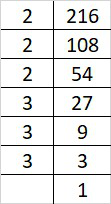216 = 2×2×2×3×3×3

By forming groups in triplet of equal factors we get, 216 = (2×2×2)×(3×3×3)

Since, 216 can be grouped into triplets of equal factors i.e (2×3) = 6

Therefore, 216 is perfect cube of 6.

(ii) 128

Prime factorization of 128 is: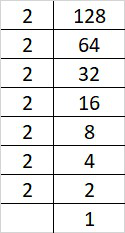128 = 2×2×2×2×2×2×2

By forming groups in triplet of equal factors we get, 128 = (2×2×2)×(2×2×2)×2

Since, 128 cannot be grouped into triplets of equal factors.

Therefore, 128 is not a perfect cube.

(iii) 1000

Prime factorization of 1000 is: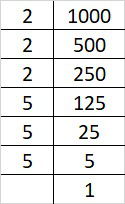1000 = 2×2×2×5×5×5

By forming groups in triplet of equal factors we get, 1000 = (2×2×2)×(5×5×5)

Since, 1000 can be grouped into triplets of equal factors i.e (2×5) = 10

Therefore, 1000 is perfect cube of 10.

(iv) 100

Prime factorization of 100 is: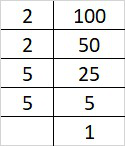100 = 2×2×5×5

Since, 100 cannot be grouped into triplets of equal factors.

Therefore, 100 is not a perfect cube.

(v) 46656

Prime factorization of 46656 is: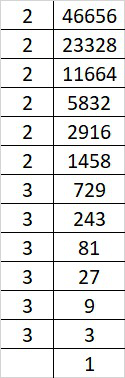46656 = 2×2×2×2×2×2×3×3×3×3×3×3

By forming groups in triplet of equal factors we get, 46656 = (2×2×2)×(2×2×2)×(3×3×3)×(3×3×3)

Since, 46656 can be grouped into triplets of equal factors i.e (2×2×3×3) = 36

Therefore, 46656 is perfect cube of 36.

### (i) 243 (ii) 256 (iii) 72 (iv) 675 (v) 100

Solution:

(i) 243

Prime factorization of 243 is: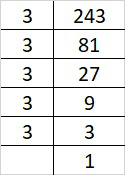243 = 3×3×3×3×3

By forming groups in triplet of equal factors we get, 243 = (3×3×3)×3×3

Since, 3 cannot form a triplets of equal factors.

Therefore, 243 must be multiplied with 3 to get a perfect cube.

(ii) 256

Prime factorization of 256 is: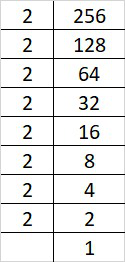256 = 2×2×2×2×2×2×2×2

By forming groups in triplet of equal factors we get, 256 = (2×2×2)×(2×2×2)×2×2

Since, 2 cannot form a triplet of equal factors.

Therefore, 256 must be multiplied with 2 to get a perfect cube.

(iii) 72

Prime factorization of 72 is: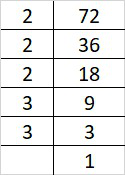72 = 2×2×2×3×3

By forming groups in triplet of equal factors we get, 72 = (2×2×2)×3×3

Since, 3 cannot form a triplet of equal factors.

Therefore, 72 must be multiplied with 3 to get perfect cube.

(iv) 675

Prime factorization of 675 is: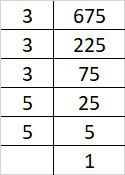675 = 3×3×3×5×5

By forming groups in triplet of equal factors we get, 675 = (3×3×3)×5×5

Since, 5 cannot form a triplet of equal factors.

Therefore, 675 must be multiplied with 5 to get perfect cube.

(v) 100

Prime factorization of 100 is:100 = 2×2×5×5

By forming groups in triplet of equal factors we get, 100 = 2×2×5×5

Since, 2 and 5 both cannot form a triplets of equal factors.

Therefore, 100 must be multiplied with 10 i.e 2×5 to get perfect cube.

### (i) 81 (ii) 128 (iii) 135 (iv) 192 (v) 704

Solution:

(i) 81

Prime factorization of 81 is: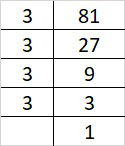81 = 3×3×3×3

By forming groups in triplet of equal factors we get, 81 = (3×3×3)×3

Since, 3 cannot form a triplets of equal factors.

Therefore, 81 must be divided by 3 to get perfect cube.

(ii) 128

Prime factorization of 128 is:128 = 2×2×2×2×2×2×2

By forming groups in triplet of equal factors we get, 128 = (2×2×2)×(2×2×2)×2

Since, 2 cannot form a triplet of equal factors.

Therefore, 128 must be divided by 2 to get perfect cube.

(iii) 135

Prime factorization of 135 is: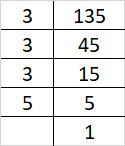135 = 3×3×3×5

By forming groups in triplet of equal factors we get, 135 = (3×3×3)×5

Since, 5 cannot form a triplet of equal factors.

Therefore, 135 must be divided by 5 to get perfect cube.

(iv) 192

Prime factorization of 192 is: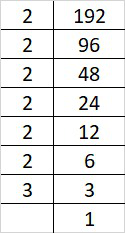192 = 2×2×2×2×2×2×3

By forming groups in triplet of equal factors we get, 192 = (2×2×2)×(2×2×2)×3

Since, 3 cannot form a triplet of equal factors.

Therefore, 192 must be divided by 3 to get perfect cube.

(v) 704

Prime factorization of 704 is: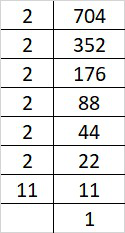704 = 2×2×2×2×2×2×11

By forming groups in triplet of equal factors we get, 704 = (2×2×2)×(2×2×2)×11

Since, 11 cannot form a triplet of equal factors.

Therefore, 704 must be divided by 11 to get perfect cube.

### Question 4: Parikshit makes a cuboid of Plasticine of sides 5 cm, 2 cm, 5 cm. How many such cuboids will he need to form a cube?

Solution:

Given: Side of cube are 5 cm, 2 cm and 5 cm.

Therefore, the volume of the cube = 5×2×5 = 50

We know that prime factorization of 50 is: 2×5×5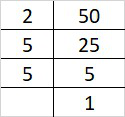Since, 2 and 5 cannot form a triplet of equal factors.

Therefore, 50 must be multiplied with 20 i.e 2×2×5 to get perfect cube.

Hence, 20 cuboid is needed to make a perfect cube.

My Personal Notes arrow_drop_up